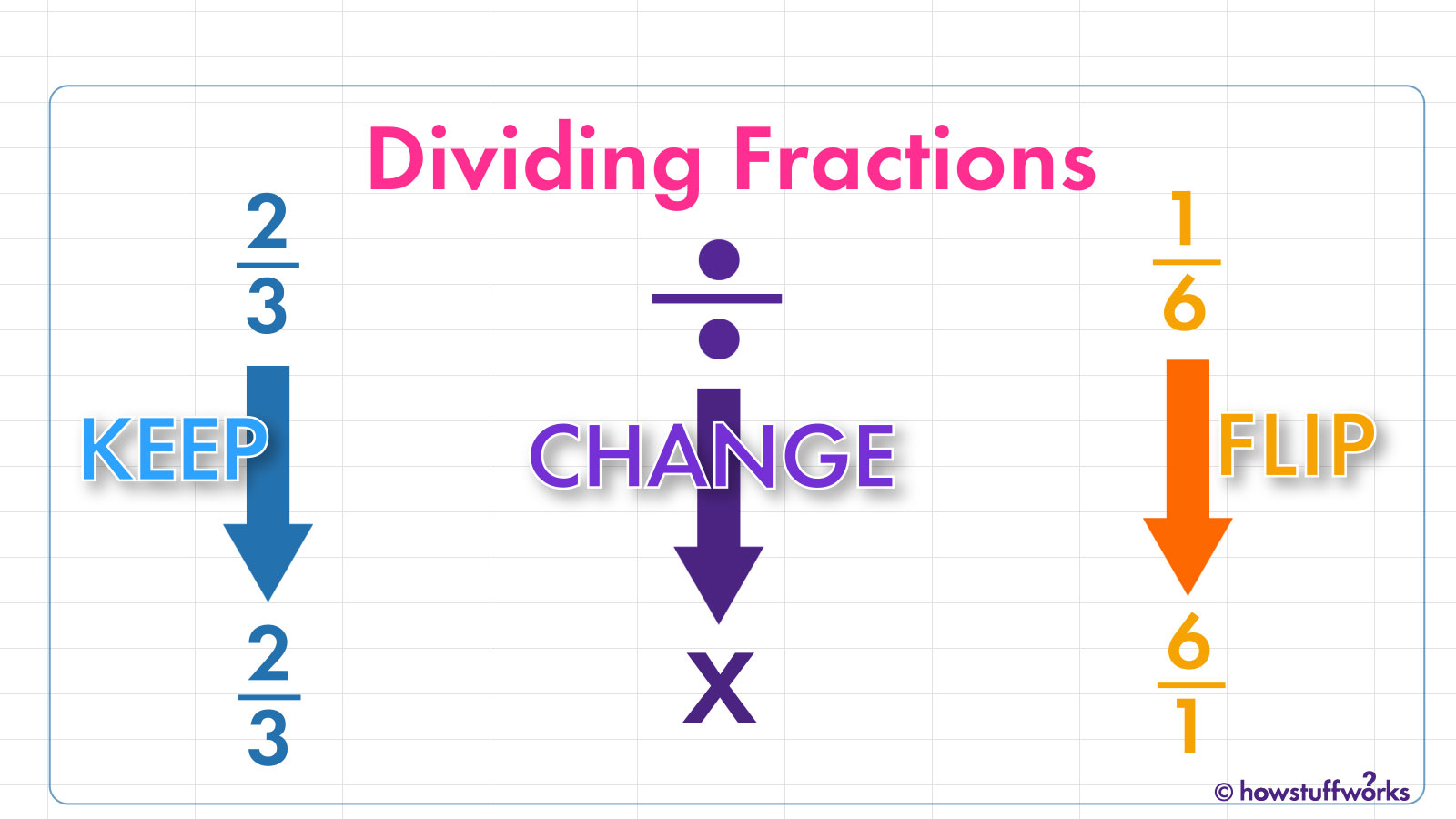# How to Divide Fractions### Step 1: Keep the Numerators and Denominators

Before you start, look at both your fractions, take a deep breath and tell yourself that if a sixth grader can learn how to divide fractions, you can become proficient at dividing fractions, too.

The first step to dividing fractions is just as simple as that little pep talk. Let’s say you’re trying to figure out the answer to 2/3 ÷ 1/6. Don’t do anything! Keep the numerator and denominator of both numbers just as they are.

### Step 2: Change the Division Sign

The second step in dividing fractions is to multiply the two fractions. So, you simply have to change the division sign (÷) sign to a multiplication sign (x): 2/3 ÷ 1/6 becomes 2/3 x 1/6.

### Step 3: Flip the Second Fraction

The third step in dividing fractions is to find the reciprocal of the divisor — but don’t panic! Dividing two fractions is the same as multiplying the first fraction by the reciprocal of the second fraction.

That just means you’ve got to flip the numerator (the top number) and the denominator (the bottom number) of the fraction on the right side of the division sign, which is called the divisor.

For instance, if you’re dividing 2/3 by 1/6, you’d start by flipping the divisor: 2/3 x 6/1 = 12/3.

### Now You Have an Improper Fraction

You might notice that the fraction is no longer in proper fraction form, in which the numerator is smaller than the denominator; it’s an improper fraction.

Improper fractions are those in which the number the fraction represents is larger than 1.

### Step 4: Is That Your Final Answer? No. Simplify the Fraction